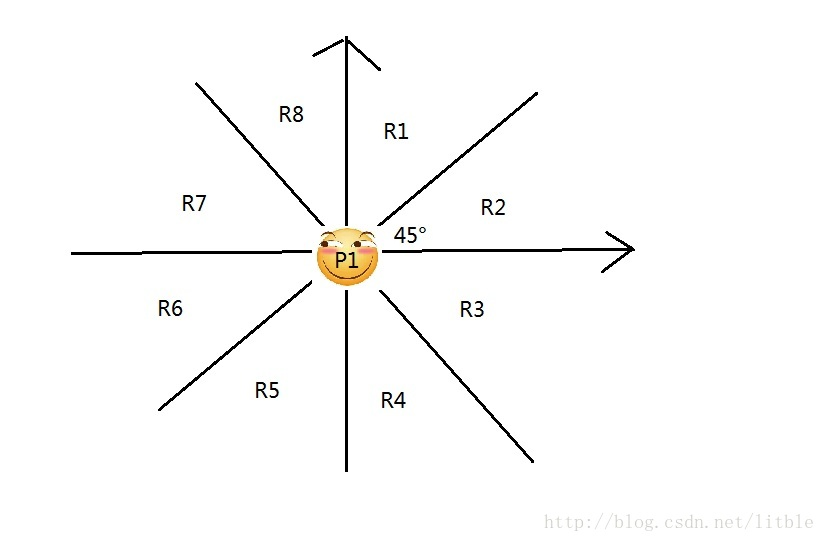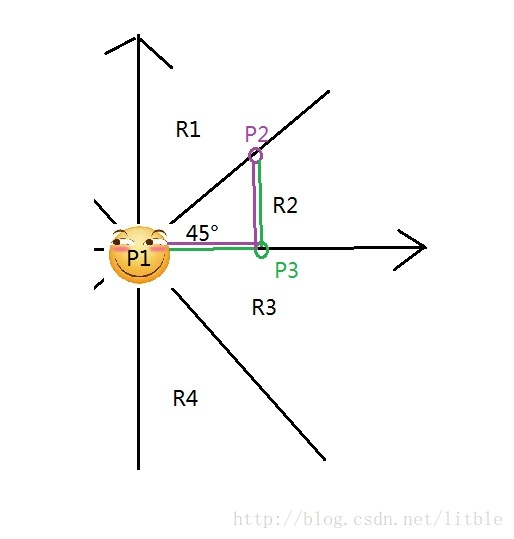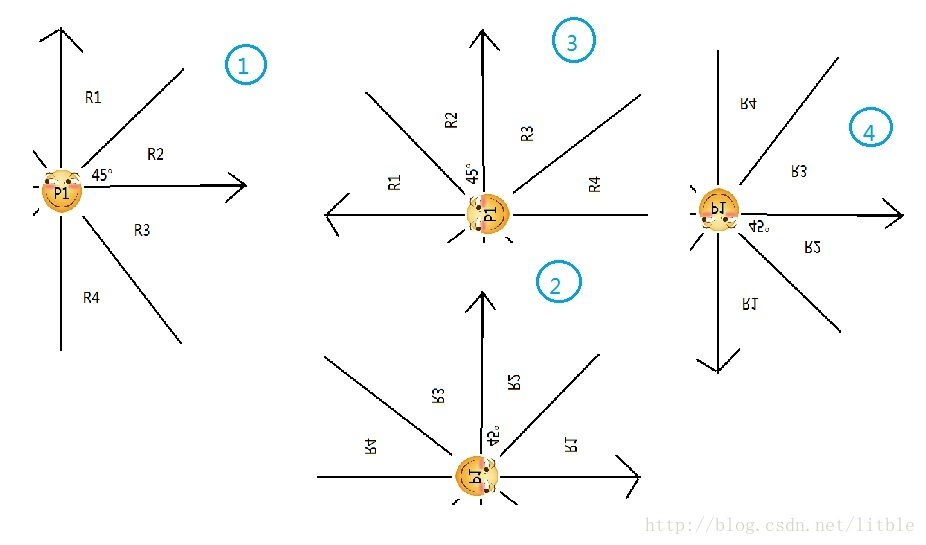# 算法分析

## 原理分析dis(指曼哈顿距离）：dis(P1,P3)+dis(P3,P2) 小于等于 dis(P1,P2)+dis(P2,P3），当且仅当如图情况时，可以取到等号。所以嘛，基佬紫的 P2 和原谅绿的 P3 显然比 P2 和滑稽脸的 P1 关系好些，你们就不要打扰它们的友谊♂啦。

## 实现分析

1. 第一次操作不搞事
2. 第二次交换 x,y 坐标
3. 第三次将 x 坐标都变成相反数
4. 第四次交换 x,y 坐标y2-y1>=x2-x1（R3,R4 区域里 y2-y1 就是负数，x2-x1 还是整数）

# 代码

（无论是时间还是长度还是空间复杂度都成功地踩了 boshi 大佬 2333）

#include<iostream>
#include<cstdio>
#include<algorithm>
#include<cstring>
#include<climits>
using namespace std;
const int N=50005;
int n,cnt,m;
int b[N],f[N],mi[N],bh[N];
struct point{int x,y,id;}p[N];
bool cmp1(point a,point b){
if(a.x==b.x)return a.y<b.y;
else return a.x<b.x;
}
struct edge{int x,y,w;}e[N<<3];
bool cmp2(edge a,edge b){return a.w<b.w;}
void init(){
int i;sort(p+1,p+1+n,cmp1);
for(i=1;i<=n;++i)
b[i]=p[i].y-p[i].x,bh[i]=-1,mi[i]=INT_MAX;
sort(b+1,b+1+n);
cnt=1;for(i=2;i<=n;++i)if(b[i]!=b[cnt])b[++cnt]=b[i];
}
int lowbit(int x){return x&(-x);}//以下是树状数组
int find(int x){
int minn=INT_MAX,re=-1;
while(x<=n){
if(mi[x]<minn)minn=mi[x],re=bh[x];
x+=lowbit(x);
}
return re;
}
void up(int x,int num,int ids){
while(x){
if(mi[x]>num)mi[x]=num,bh[x]=ids;
x-=lowbit(x);
}
}
int dis(int a,int b){return abs(p[a].x-p[b].x)+abs(p[a].y-p[b].y);}
int ff(int x){//并查集查找操作
if(f[x]==x)return x;
f[x]=ff(f[x]);return f[x];
}
void kurscal(){//最小生成树
int i,r1,r2,ans=0,js=0;
for(i=1;i<=n;++i)f[i]=i;
sort(e+1,e+1+m,cmp2);
for(i=1;i<=m;++i){
r1=ff(e[i].x),r2=ff(e[i].y);
if(r1!=r2)f[r1]=r2,ans+=e[i].w,++js;
if(js==n-1)break;
}
printf("%d",ans);
}
int main(){
int i,cas,kl,x;
scanf("%d",&n);
for(i=1;i<=n;++i)
scanf("%d%d",&p[i].x,&p[i].y),p[i].id=i;
for(cas=1;cas<=4;++cas){
if(cas==2||cas==4)
for(i=1;i<=n;++i)swap(p[i].x,p[i].y);
else if(cas==3)
for(i=1;i<=n;++i)p[i].x=-p[i].x;
init();
for(i=n;i>=1;--i){
kl=lower_bound(b+1,b+1+cnt,p[i].y-p[i].x)-b;
x=find(kl);
up(kl,p[i].x+p[i].y,i);
}
}
kurscal();
return 0;
}


### 1 条评论